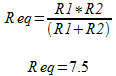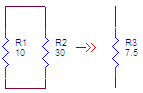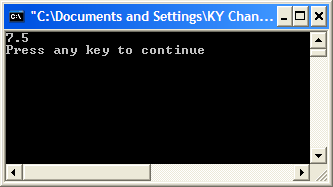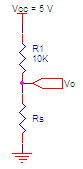The following information is for the EMANT300, EMANT380

# Exercise 3 – Variables, Expressions & Statements

## Objective

• Variables

• Expressions

• Statements

We will view and run a program to calculate the equivalent resistance of two resistors in parallel. When you have two resistors in parallel, the equivalent resistance is given by1.Open the Visual Basic solution VB_2010_Solution.sln. A Visual Basic Solution file has the extension .sln and contains a static list of one or more projects.

2. Set the Variables project as the startup project.

3. View the project code by opening the project's Module1.vb## Program 3.1 Calculate Equivalent Resistance

``````Module Module1
Sub Main()
Dim R1, R2, Req As Double
R1 = 10
R2 = 30
Req = R1 * R2 / (R1 + R2)
Console.WriteLine(Req)
End Sub
End Module
``````

## Variable Declaration

``Dim R1, R2, Req As Double``

• R1, R2 and Req are called variables and they hold values in the computer memory.

• A variable declaration gives it a name and the data type (in this case double). A declaration begins with the keyword Dim

• A variable must be declared before using it.

## Data Types

• The eight integral types provide support for 8-bit, 16-bit, 32-bit, and 64-bit values in signed or unsigned form.

• The two floating point types, float and double, are represented using the 32-bit single-precision and 64-bit double-precision IEEE 754 formats.

• The decimal type is a 128-bit data type suitable for financial and monetary calculations.

• Visual Basic’s bool type is used to represent boolean values—values that are either true or false.

• Character and string processing in Visual Basic uses Unicode encoding. The char type represents a 16-bit Unicode code unit, and the string type represents a sequence of 16-bit Unicode code units.

The following table summarizes Visual Basic’s numeric types.

 Category Bits Type Range/Precision Signed integral 16 Short –32,768...32,767 32 Integer –2,147,483,648...2,147,483,647 64 Long –9,223,372,036,854,775,808...9,223,372,036,854,775,807 Unsigned integral 8 Byte 0...255 Floating point 64 Double 5.0×10−324 to 1.7×10308, 15-digit precision Decimal 128 Decimal 1.0×10−28 to 7.9×1028, 28-digit precision

## Assignment of Values into Variables

Once we have declared our variables, we can place values in them by way of the assignment operator =

``````R1 = 10
R2 = 30
``````

R1 now holds 10.0 and R2 holds 30.0

The general form of the assignment statement is

variable = expression

## Expressions

Expressions are constructed from operands and operators. The operators of an expression indicate which operations to apply to the operands.

• Examples of operators include +, -, *, /.

• Examples of operands include literals, fields, local variables, and expressions.

When an expression contains multiple operators, the precedence of the operators controls the order in which the individual operators are evaluated. For example, the expression (R1 + R2) is evaluated first because the ( ) operator has higher precedence than the * or / operators.

``````R1 * R2 / (R1 + R2)
``````

The following table summarizes arithmetic Visual Basic’s operators, listing the operator categories in order of precedence from highest to lowest. Operators in the same category have equal precedence.

 Category Expression Description Multiplicative x * y Multiplication x / y Division (float) i \ j Division (integer) x Mod y Modulus Additive x + y Addition, string concatenation, delegate combination x – y Subtraction, delegate removal Assignment x = y Assignment

## Statements

The actions of a program are expressed using statements. Visual Basic supports several different kinds of statements, a number of which are defined in terms of embedded statements.

• Declaration statements are used to declare local variables and constants.

• Expression statements are used to evaluate expressions. Expressions that can be used as statements include method invocations, object allocations using the New operator, assignments using = and the compound assignment operators.

``````Req = R1 * R2 / (R1 + R2);
``````

## Using Integral Data Types

Integral variables like Integer hold whole numbers like 10, 20, 7 compared to floating point variables like Double which can hold values like 10.1, 7.5

1. Replace

``Dim R1, R2, Req As Double``

with

``Dim R1, R2, Req As Integer``

## Program 3.2 Calculate Equivalent Resistance using Integral Data Type

``````
Module Module1
Sub Main()
Dim R1, R2, Req As Integer
R1 = 10
R2 = 30
Req = R1 * R2 \ (R1 + R2)
Console.WriteLine(Req)
End Sub
End Module
``````

1. Run the program again. The result shows 7 rather than 7.5 because the answer is truncated. So be careful when selecting the data type for your variables to prevent errors.## Explicit Casting

``````Dim R1 As Double
Dim R2 As Integer
R1 = 10.5
R2 = R1
``````

If you add the following line to the top of your source code

``````Option Strict On
``````

the following line will report a compilation error because you try to assign a Double to an Integer.

``````R2 = R1
``````

To avoid the compilation error, use casting where the term CType(expression, typename) tells the compiler to convert the value within into an integer value. However, it will still result in a loss of data - R2 is 10 instead of 10.5. You can use this type of conversion to convert any numeric data types.

``````R2 = CType(R1, Integer)
``````

## Measure resistanceData Acquisition Cards normally measure only voltages. Often it is necessary to measure resistances if the sensor used changes its resistance with respect to the physical phenomenal measured.

Examples include the Light Dependent Resistor where the resistance changes with Light Intensity and the Thermistor where resistance changes with temperature. One simple solution is to connect a resistor in series with the sensor.The following Visual Basic code calculates the sensor resistance given the voltage across the sensor is 3.0 V.

``````Dim R1, Rs, Vcc, Vo As Double
R1 = 10000.0
Vo = 3.0
Vcc = 5.0
Rs = (R1 * Vo) / (Vcc - Vo)
Console.WriteLine(Rs)
``````